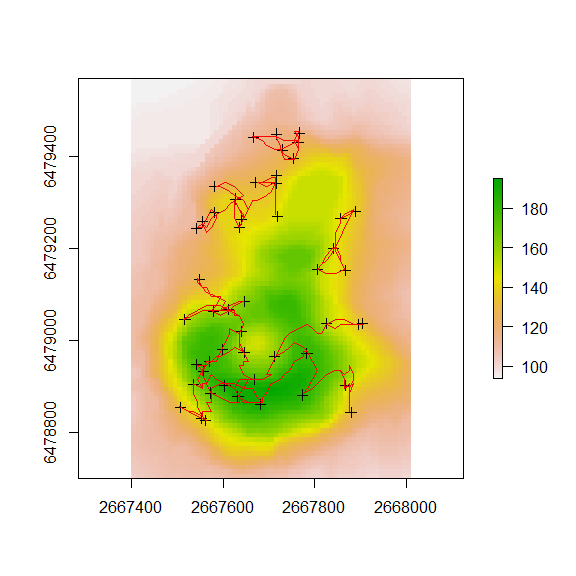# 1. Introduction

This vignette describes some examples using the create_lcp_network function in the leastcostpath package.

The leastcostpath package is written for use in the R environment (R Core Team, 2016). It provides functionality to calculate Least Cost Paths using multiple cost functions that approximate the difficulty of moving across a landscape, taking into account obstacles and local fricion (e.g. slope). Furthermore, this package allows for the incorporation of cost when traversing across slope, as well as other factors such as landscape features.

The create_lcp_network function uses a specified matrix to assess which locations to calculate the least cost path between.

# 2. Setup

library(rgdal)
library(rgeos)
library(sp)
library(raster)
library(spdep)
library(gdistance)
library(leastcostpath)
r <- raster::raster(system.file('external/maungawhau.grd', package = 'gdistance'))

locs <- sp::spsample(gBuffer(as(extent(r), "SpatialPolygons"), width = -100),n=50,'random')

final_cs <- create_slope_cs(dem = r, cost_function = 'tobler', neighbours = 16) %>%
"*" (create_traversal_cs(dem = r, neighbours = 16))

plot(r)
plot(locs, add = T)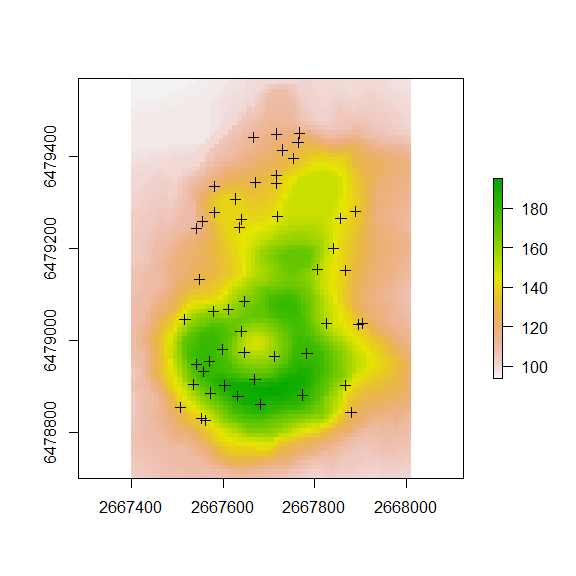# 3. Example 1: Least Cost Path Network - User specified matrix

The below matrix specifies that we want to calculate least cost paths between the following locations:

• Location 1 to 2
• Location 4 to 2
• Location 2 to 4
• Location 1 to 3
locs_matrix <- cbind(c(1, 4, 2, 1), c(2, 2, 4, 3))

locs_matrix
##      [,1] [,2]
## [1,]    1    2
## [2,]    4    2
## [3,]    2    4
## [4,]    1    3
 lcp_network <- final_cs %>%
create_lcp_network(., locations = locs, nb_matrix = locs_matrix, cost_distance = FALSE, parallel = FALSE)

plot(r)
plot(lcp_network, add = T, col = "red")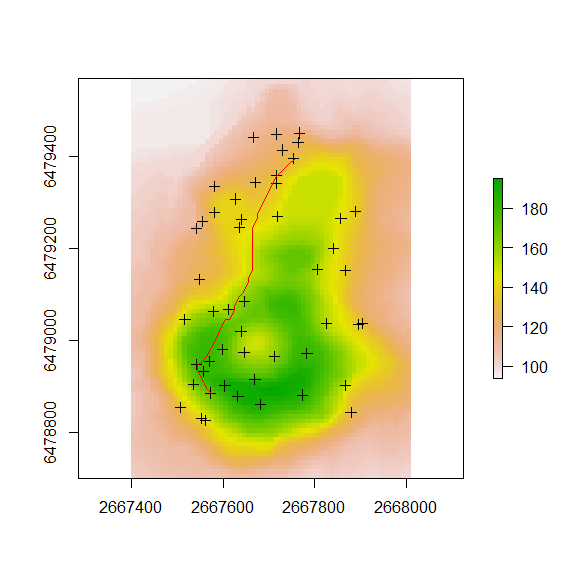# 4. Example 2: Least Cost Path Network - Delauney Network

neighbour_pts <- spdep::tri2nb(locs)
##
##      PLEASE NOTE:  The components "delsgs" and "summary" of the
##  object returned by deldir() are now DATA FRAMES rather than
##  matrices (as they were prior to release 0.0-18).
##  See help("deldir").
##
##      PLEASE NOTE: The process that deldir() uses for determining
##  duplicated points has changed from that used in version
##  0.0-9 of this package (and previously). See help("deldir").
origin_ids <- base::rep(base::seq_along(neighbour_pts), base::sapply(neighbour_pts, function(x) base::length(x)))

destination_ids <- base::unlist(neighbour_pts)

locs_matrix <- base::cbind(origin_ids, destination_ids)

head(locs_matrix)
##      origin_ids destination_ids
## [1,]          1               3
## [2,]          1              17
## [3,]          1              20
## [4,]          1              21
## [5,]          1              38
## [6,]          1              50
tail(locs_matrix)
##        origin_ids destination_ids
## [265,]         49              46
## [266,]         50               1
## [267,]         50              14
## [268,]         50              16
## [269,]         50              21
## [270,]         50              38
 lcp_network <- final_cs %>%
create_lcp_network(., locations = locs, nb_matrix = locs_matrix, cost_distance = FALSE, parallel = FALSE)

plot(r)
plot(lcp_network, add = T, col = "red")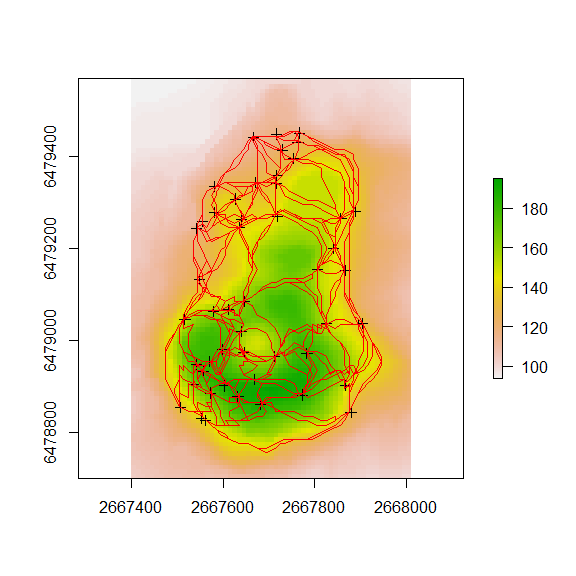# 5. Example 3: Least Cost Path Network - Gabriel Network

neighbour_pts <- spdep::gabrielneigh(locs)

locs_matrix <- base::cbind(neighbour_pts$from, neighbour_pts$to)

head(locs_matrix)
##      [,1] [,2]
## [1,]    1    3
## [2,]    1   17
## [3,]    1   20
## [4,]    1   50
## [5,]    2   12
## [6,]    2   13
tail(locs_matrix)
##       [,1] [,2]
## [83,]   39   45
## [84,]   39   46
## [85,]   40   43
## [86,]   41   43
## [87,]   41   48
## [88,]   44   46
 lcp_network <- final_cs %>%
create_lcp_network(., locations = locs, nb_matrix = locs_matrix, cost_distance = FALSE, parallel = FALSE)

plot(r)
plot(lcp_network, add = T, col = "red")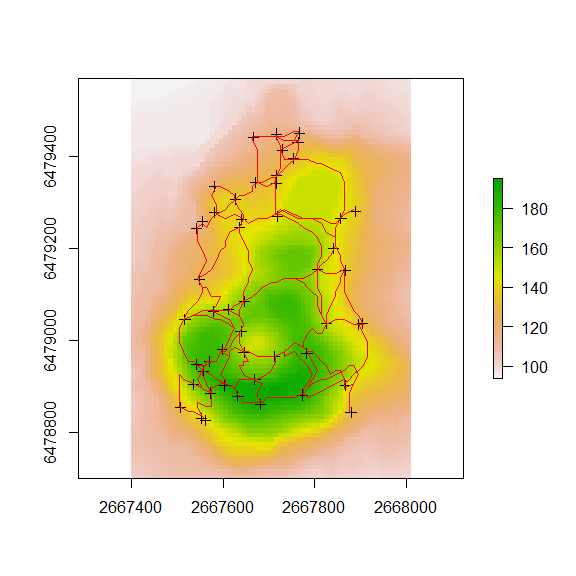# 6. Example 4: Least Cost Path Network - K Nearest Network

neighbour_pts <- spdep::knearneigh(locs, k= 2)

k_network <- function(k_neigh) {

k_neigh <- k_neigh$nn col_no <- ncol(k_neigh) locs <- seq_along(1:nrow(neighbour_pts$nn))

k1 <- cbind(locs, k_neigh[, 1])

if (col_no > 1) {

print("greater than 1")

k <- list()

for (i in 2:col_no) {
k[[i]] <- k_neigh[, c(1, i)]

}

knear <- do.call(rbind, k)

kplus <- rbind(k1, knear)

return(kplus)

} else {

print("less than 1")

return(k1)

}

}

neighbour_pts <- k_network(neighbour_pts)
##  "greater than 1"
locs_matrix <- neighbour_pts

head(locs_matrix)
##      locs
## [1,]    1 17
## [2,]    2 48
## [3,]    3 21
## [4,]    4 24
## [5,]    5 25
## [6,]    6 14
tail(locs_matrix)
##        locs
##  [95,]    7 39
##  [96,]   39 44
##  [97,]   11 13
##  [98,]    2 12
##  [99,]   11 28
## [100,]   38 14
 lcp_network <- final_cs %>%
create_lcp_network(., locations = locs, nb_matrix = locs_matrix, cost_distance = FALSE, parallel = FALSE)

plot(r)
plot(lcp_network, add = T, col = "red")